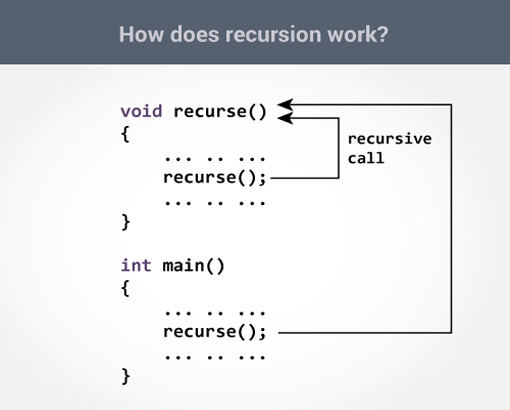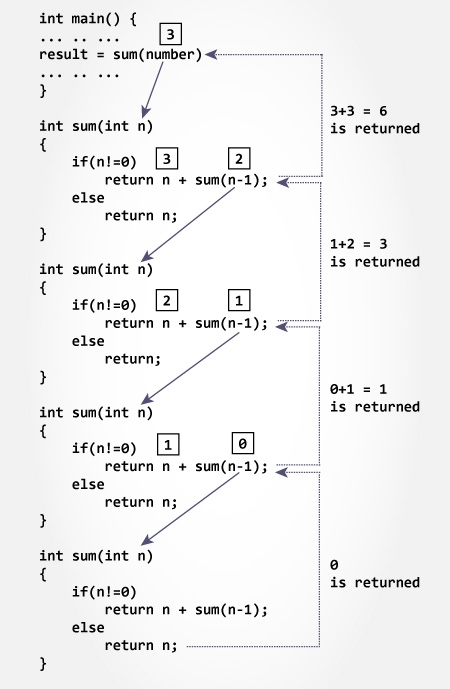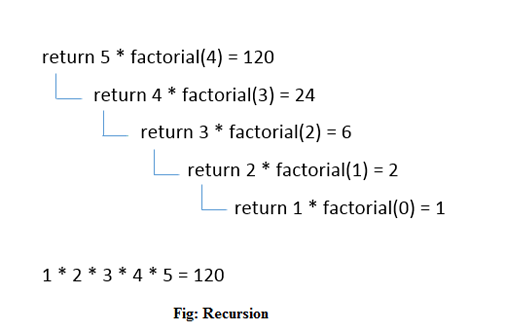# Java Tutorial

## Recursion in Java

A method that calls itself is known as a recursive method. And, this technique is known as recursion.

##### How recursion works?In the above program, recurse() method is called from inside the main method at first (normal method call).

Also, recurse() method is called from inside the same method, recurse(). This is a recursive call.

The recursion continues until some condition is met to prevent it from execution. If not, infinite recursion occurs.

Hence, to prevent infinite recursion, if...else statement (or similar approach) can be used where one branch makes the recursive call and other doesn't.

##### Syntax:
```
returntype methodname(){
//code to be executed
methodname();//calling same method
}
```
##### Java Recursion Example : Infinite times
```
public class RecursionExample1 {
static void p(){
System.out.println("hello");
p();
}

public static void main(String[] args) {
p();
}
}
```

##### Output:
```
hello
hello
...
java.lang.StackOverflowError
```
##### Java Recursion Example : Finite times
```
public class RecursionExample2 {
static int count=0;
static void p(){
count++;
if(count<=5){
System.out.println("hello "+count);
p();
}
}
public static void main(String[] args) {
p();
}
}
```

```
hello 1
hello 2
hello 3
hello 4
hello 5
```
##### Java Recursion Example : Factorial Number
```
public class RecursionExample3 {
static int factorial(int n){
if (n == 1)
return 1;
else
return(n * factorial(n-1));
}

public static void main(String[] args) {
System.out.println("Factorial of 5 is: "+factorial(5));
}
}
```

##### Output:
```
Factorial of 5 is: 120

```
##### Working of above program:

Initially, factorial() is called from the main() method with number passed as an argument.

Inside factorial() method, the value of n is 4 initially. During the next recursive call, 3 is passed to the factorial() method. This process continues until n is equal to 0.

When n is equal to 0, if condition fails and the else part is executed which returns 1, and accumulated result is passed to the main() method.

```
factorial(5)
factorial(4)
factorial(3)
factorial(2)
factorial(1)
return 1
return 2*1 = 2
return 3*2 = 6
return 4*6 = 24
return 5*24 = 120
```##### Java Recursion Example : Fibonacci Series
```
public class RecursionExample4 {
static int n1=0,n2=1,n3=0;
static void printFibo(int count){
if(count>0){
n3 = n1 + n2;
n1 = n2;
n2 = n3;
System.out.print(" "+n3);
printFibo(count-1);
}
}

public static void main(String[] args) {
int count=14;
System.out.print(n1+" "+n2);//printing 0 and 1
printFibo(count-2);//n-2 because 2 numbers are already printed
}
}
```

##### Output:
```

0 1 1 2 3 5 8 13 21 34 55 89 144 233

```